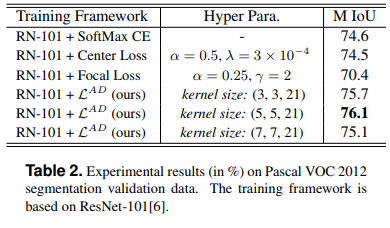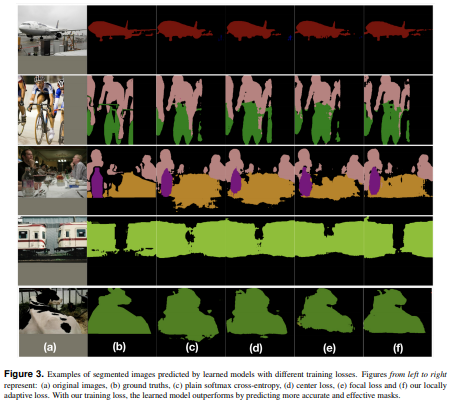The authors propose a novel loss for multi-class segmentation. The core idea behind their loss is to force the network to minimize the overall loss per class rather than per point (as in a standard CE loss).

The Locally Adaptive Loss is really simple. As we see in fig. 2, they pool scores from neighboring pixels with the same class to get an average feature vector. This is called the Selective Pooling.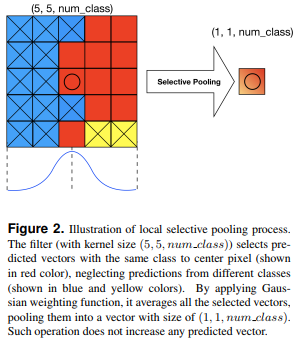This feature vector can then be fed to a standard cross-entropy after being normalized (eq. 4).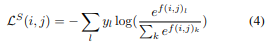The final loss is the Minkowski normalization with all valid patches. As $$k$$ increases, the difficult areas are highlighted. The authors argue that this helps in segmentation since there is often classes with only few samples. They found that $$k=5$$ gives the best results on Pascal VOC 2012.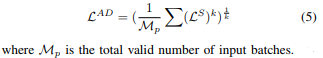## Results